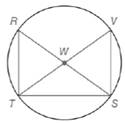Chapter 6.1, Problem 32E### Elementary Geometry for College St...

6th Edition
Daniel C. Alexander + 1 other
ISBN: 9781285195698

#### Solutions

Chapter
Section### Elementary Geometry for College St...

6th Edition
Daniel C. Alexander + 1 other
ISBN: 9781285195698
Textbook Problem
1 views

# In Exercises 32 to 37, write a paragraph proof. Given: R S ¯  and  T V ¯  are diameters of  ⊙ W Prove: Δ R S T ≅ Δ V T STo determine

To prove:

A paragraph proof when RS¯ and TV¯ are diameters of W then ΔRSTΔVTS.

Explanation

Given:

The line segments RS¯ and TV¯ are diameters of W.

Rule used:

SAS congruency rule:

If two sides and the included angle of one triangle are equal to two sides and included angle of another triangle, then the triangles are congruent.

SSS congruency rule:

If three sides of one triangle are equal to three sides of another triangle then the triangles are congruent.

Proof:

Let RS¯ and TV¯ be the diameters of circle W.

Since all the diameters of the circle are of same length, RS¯TV¯.

Also, all the radii of the circle are of same length, WR¯WV¯ and WT¯WS¯.

From the figure, we see that the diameters RS¯ and TV¯ form vertical angles, which are congruent

### Still sussing out bartleby?

Check out a sample textbook solution.

See a sample solution

#### The Solution to Your Study Problems

Bartleby provides explanations to thousands of textbook problems written by our experts, many with advanced degrees!

Get Started

#### Find more solutions based on key concepts